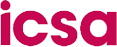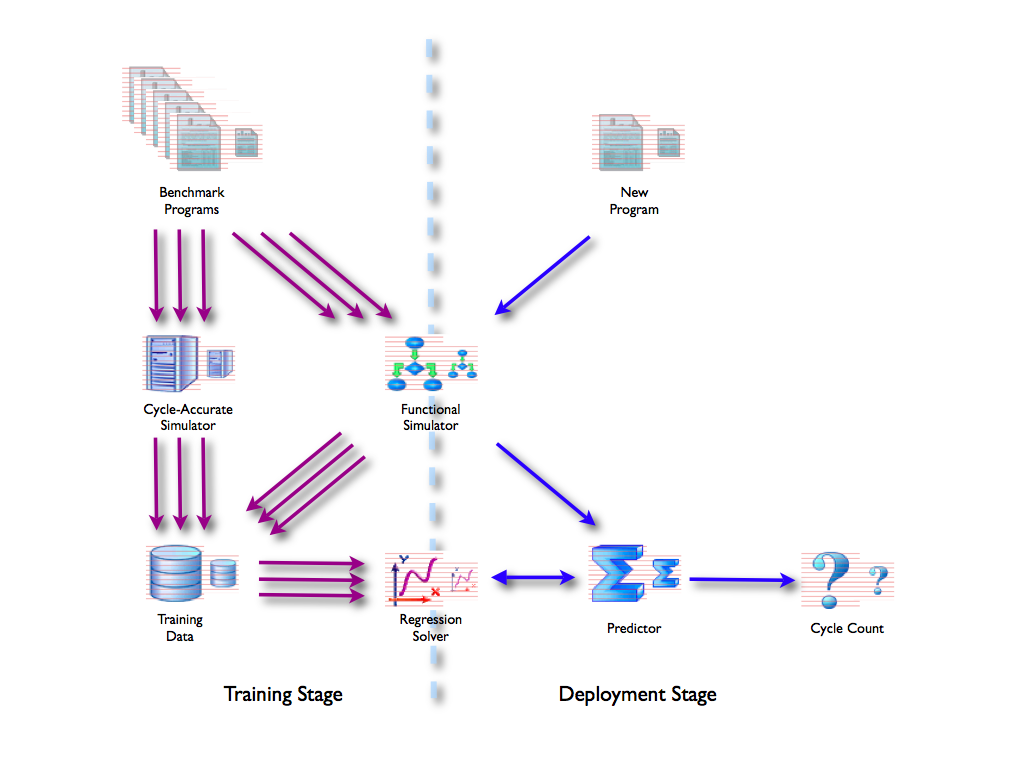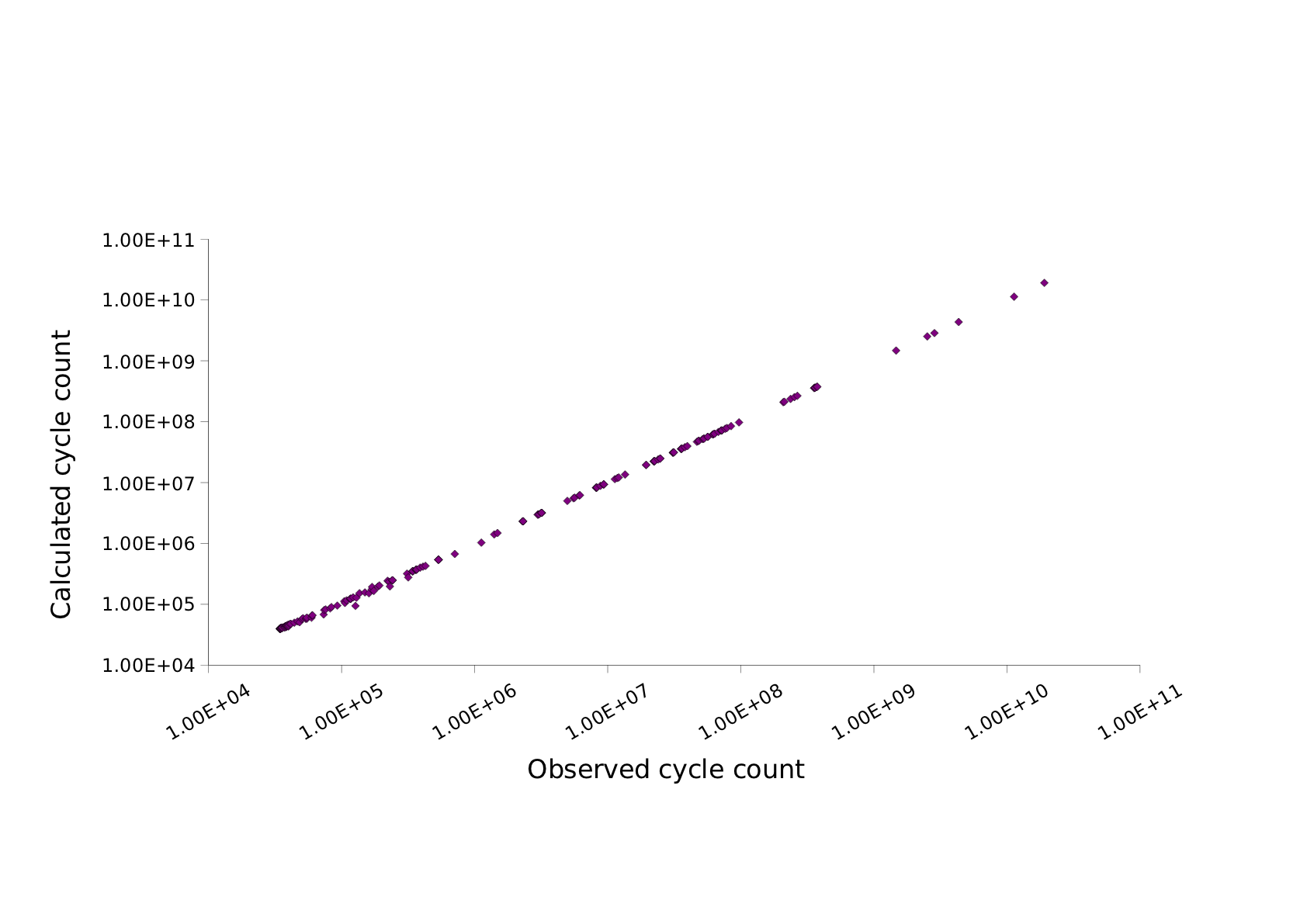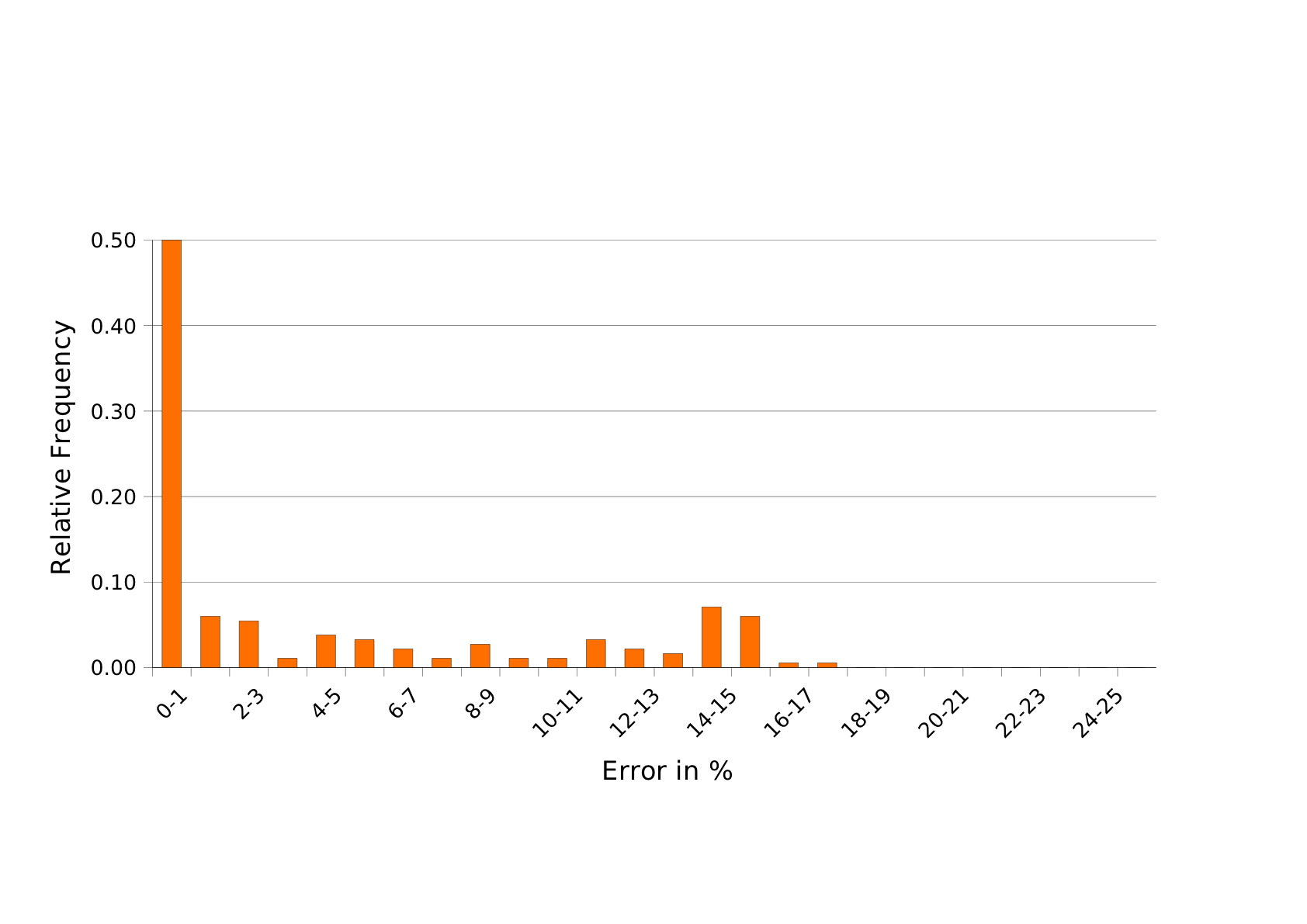Institute for Computing Systems Architecture

Instruction set simulators are indispensable tools in both ASIP design space exploration and the software development and optimisation process for existing platforms. Despite the recent progress in improving the speed of functional instruction set simulators cycle-accurate simulation is still prohibitively slow for all but the most simple programs. This severely limits the applicability of cycle-accurate simulators in the performance evaluation of complex embedded applications. In the PASTA project we explore a novel approach, namely the prediction of cycle counts based on information gathered during fast functional simulation and prior training. We have evaluated our approach against a cycle-accurate ARM v5 architecture simulator and a set of approx. 300 benchmarks. We demonstrate it is capability of providing highly accurate performance predictions with an average error of less than 5.8% at a fraction of the time for cycle-accurate simulation.

#### OverviewCycle-approximate instruction set simulation (ISS) is based on a two-staged approach to constructing a performance predictor: A Training Stage in which a set of training/benchmarking programs are profiled and a later Deployment Stage, where the performance of previously unseen programs is predicted. An illustration of these two stages is shown in the above diagram. During the training stage a set of benchmark applications is executed and profiled on both the cycle-accurate and the functional ISS. For each program the exact cycle count and the values of the various counters maintained in the functional ISS are collected and together they form a single data point (y, x). As soon as this data is available for all programs in the training set the Regression Solver calculates the regression coefficients according to a predefined regression model and stores them for later use. On entering the deployment stage the cycle-accurate simulator is not used any more, but all simulations are performed by the functional simulator. As before, this simulator is used to generate a characteristic profile, i.e. the vector x, of the program under examination. The regression model with the previously calculated coefficients is then used as a predictor and evaluated at the point x, resulting in the predicted cycle count y for the new program.

#### Results

The selection of an appropriate regression model (linear, polynomial, various non-linear functions etc.) is a design parameter and has a critical impact on how well the regression function can describe the observed data. Our hypothesis is that the cycle count is a near-linear function in the various counters maintained by the ISS. In order to test this hypothesis we compare the predicted cycle counts y* with observed cycle counts y performing regression over the entire data set (all programs and all counters except the observed cycle count).The above graph shows the close match between the observed and calculated cycle counts. The data points are concentrated near the ideal straight line with only a very few exceptional outliers, especially at the lower end of the scale. We have calculated the residuals and have found an average error of 4.5%.A more detailed breakdown of the distribution of errors is shown in the second graph, where the relative error frequency is plotted in a histogram against the percentage error interval. This diagram shows that the vast majority of programs can be described with a very small error. In fact, for about 50% of all programs the error is less than 1%. For 75% of all programs the error is less than 8% and only three programs have an error of 15%. The maximum error, however, is relatively large with a value of 26.1%.